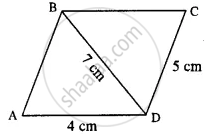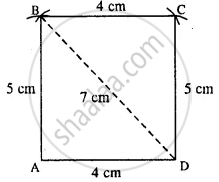# Construct a Parallelogram Abcd, If : Ad = 4 Cm, Dc = 5 Cm and Diagonal Bd = 7 Cm. - Mathematics

Sum

Construct a parallelogram ABCD, if :

AD = 4 cm, DC = 5 cm and diagonal BD = 7 cm.

#### Solution

The rough figure is as follow :∵ opposite sides of ||gm are equal

∴ AB = DC = 5 cm

Actual ||gm is constructed as followSteps :

1. Draw AD = 4 cm.
2. From A, draw an arc of radius 5 cm.
3. From B, draw an arc of radius 4 cm.
4. From D, draw an arc of radius 5 cm which intersects the first arc at C.
5. Join AB, BD, BC, and CD.
Thus ABCD is the required || gm.
Concept: Construction of Parallelograms
Is there an error in this question or solution?

#### APPEARS IN

Selina Concise Mathematics Class 8 ICSE
Chapter 18 Constructions
Exercise 18 (D) | Q 2.3 | Page 211